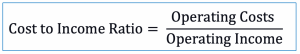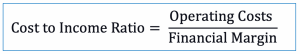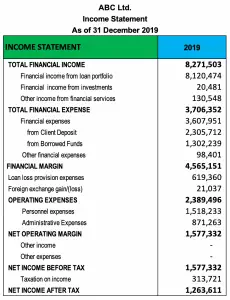# Cost to Income Ratio

## Overview

Cost to income ratio is the measurement that is used in the company in order to evaluate its efficiency. It is usually used in the microfinance institution or bank in order to measure its operating cost compared to the income it generates.

In order to have a better analysis of the company’s performance in terms of efficiency, the microfinance institution or bank (the company) may need to benchmark the ratio to the historical period or the industry average.

The lower the cost to income ratio is the better it is for the company’s performance. Likewise, the lower the ratio is, the more efficiency the company can achieve in the period.

In this case, in order to reduce cost to income, the company needs to either increase its operating income or reduce its operating costs. Operating costs, in this case, include both personnel expenses and administration expenses.

## Cost to Income Ratio Formula

We can calculate cost to income ratio with the formula of using the operating costs or expenses to divide with the operating income.It is useful to note that there are usually financial costs or expenses. In this case, operating costs should not include financial expenses.

Also, in the bank or microfinance institution, operating income is usually the result of the deduction of financial expenses from the financial income.

Hence, as the financial income minus financial expenses equal to the financial margin, the operating income here is usually replaced by the financial margin. Likewise, the cost to income ratio formula in bank or microfinance institution may be changed to as below:***Financial margin = Financial income – Financial expenses

## Cost to Income Ratio Example

For example, ABC Ltd. which is a small bank has the income statement as of 31 December 2019 as below:Calculate cost to income ratio for the period of 2019

Solution

With the cost to income ratio formula above, we can calculate as below:

Operating costs = USD 2,389,496

Financial income = USD 8,271,503

Financial expenses = USD 3,706,352

Operating income or financial margin = 8,271,503 – 3,706,352 = USD 4,565,151

Cost to Income Ratio = 2,389,496/ 4,565,151 = 52.34%

This means that in operating the company, ABC Ltd. spent 52.34% to generate its operating income which is considered good. However, to be more accurate in measuring the company’s efficiency, we may need to use the last period figure or the industry average. If this result is lower than the last period or the industry average, the company’s performance will be clearly better.

The excel calculation and data in the picture of the example above can be found in the link here: Cost to income ratio calculation excel

## Cost to Income Ratio and Profitability

Cost to income ratio measures the costs that are necessary to generate income. This gives the result that the lower the ratio is the better profitability the company may achieve.

In this case, when we compare the two, the cost to income and profitability ratio (e.g. profit margin, return on assets, or return on equity), they are going the opposite ways.

For example, when the cost to income ratio is higher than the last period, it means the company’s performance is worse (in term of efficiency). On the other hand, the higher the profitability ratio is, the better the company performs.

In summary, the cost to income ratio is a type of efficiency ratio that is usually used in bank or microfinance institution. The lower it is the better it would be for the company as it would show that the company needs only small costs to generate the operating income.